## 2.1.1 POISSON's Equation

By introducing a vector potential and a scalar potential MAXWELL's equations can often be rewritten in a more practical form. The vector potential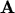is defined by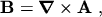(2.7)

which fulfills eqn. (2.2) since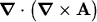evaluates to zero for every vector field. Inserting eqn. (2.7) into eqn. (2.1) gives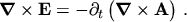(2.8)

Interchanging the order of the time derivative and the curl operator,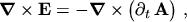(2.9)

and using the associative property of the curl operator,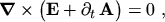(2.10)

the argument of the curl operator can be substituted by the gradient of a scalar potential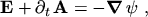(2.11)

since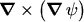yields zero for every scalar field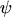. The minus sign on the right hand side of eqn. (2.11) is introduced by convention based on historical reasons.

In the quasi-stationary case, which holds true for semiconductor devices2.1, the time derivative of the vector potential can be neglected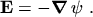(2.12)

POISSON's equation is finally obtained by inserting eqn. (2.12) into eqn. (2.5)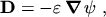(2.13)

which is in turn inserted into eqn. (2.4)(2.14)

In the case of vanishing space charge density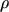POISSON's equation simplifies to the LAPLACE equation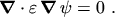(2.15)

M. Gritsch: Numerical Modeling of Silicon-on-Insulator MOSFETs PDF